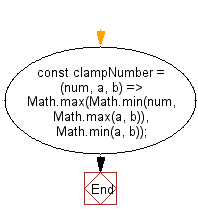# JavaScript: Clamp a number within the inclusive range specified by the given boundary values a and b

## JavaScript fundamental (ES6 Syntax): Exercise-266 with Solution

Write a JavaScript program to clamp a number within the inclusive range specified by the given boundary values a and b.

• If num falls within the range, return num.
• Otherwise, return the nearest number in the range.

Sample Solution:

JavaScript Code:

``````//#Source https://bit.ly/2neWfJ2
const clampNumber = (num, a, b) => Math.max(Math.min(num, Math.max(a, b)), Math.min(a, b));
console.log(clampNumber(2, 3, 5));
console.log(clampNumber(1, -1, -5));
```
```

Sample Output:

```3
-1
```

Flowchart:Live Demo:

See the Pen javascript-basic-exercise-266-1 by w3resource (@w3resource) on CodePen.

Improve this sample solution and post your code through Disqus

What is the difficulty level of this exercise?

Test your Programming skills with w3resource's quiz.

﻿

## JavaScript: Tips of the Day

Returns the difference (in days) between two dates

Example:

```const tips_DiffBetweenDates = (dateInitial, dateFinal) =>
(dateFinal - dateInitial) / (1000 * 3600 * 24);
console.log(tips_DiffBetweenDates(new Date('2020-05-13'), new Date('2020-06-05')));
```

Output:

```23
```